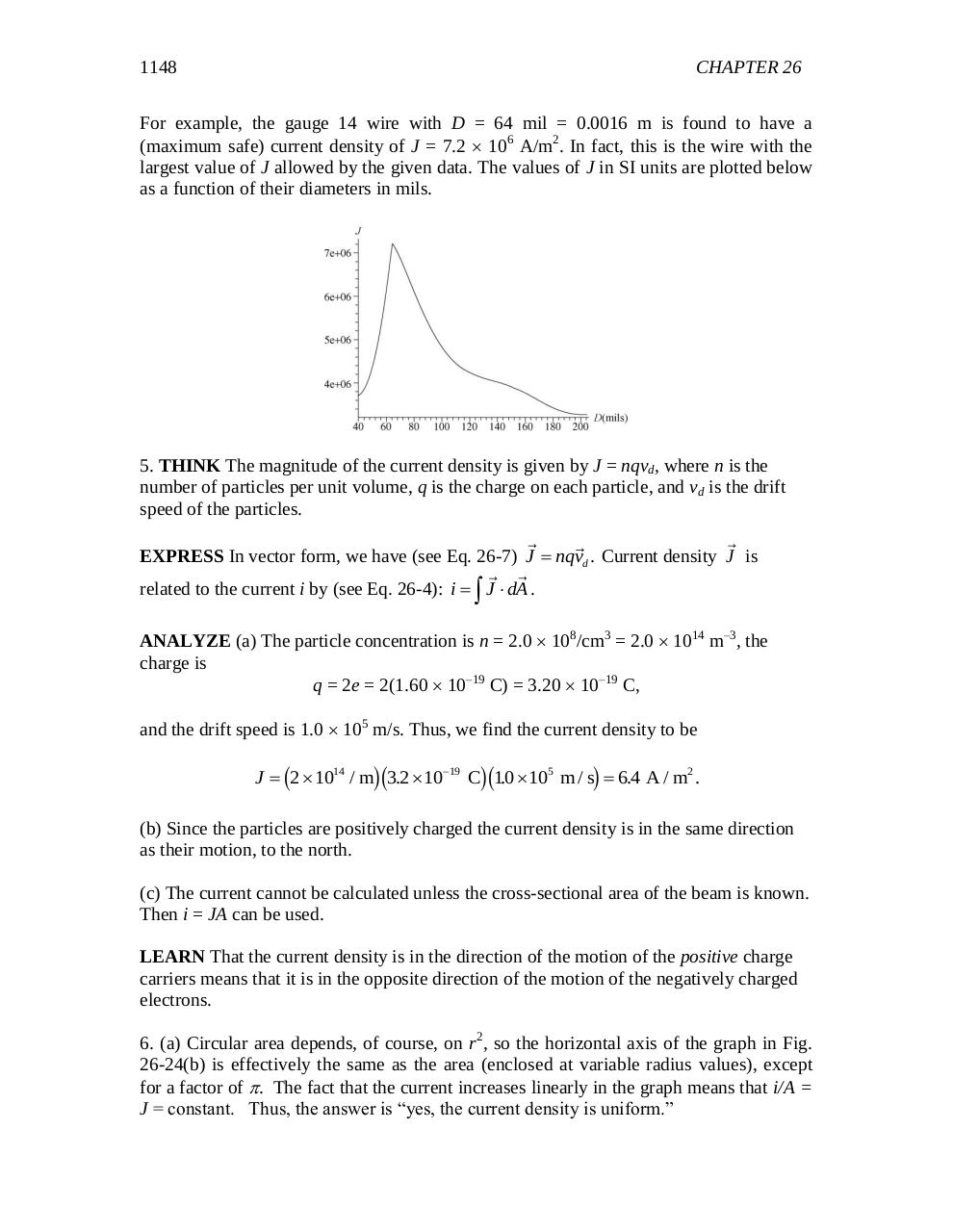# chapter 26.pdfPage 1 23426

#### Text preview

1148

CHAPTER 26

For example, the gauge 14 wire with D = 64 mil = 0.0016 m is found to have a
(maximum safe) current density of J = 7.2  106 A/m2. In fact, this is the wire with the
largest value of J allowed by the given data. The values of J in SI units are plotted below
as a function of their diameters in mils.

5. THINK The magnitude of the current density is given by J = nqvd, where n is the
number of particles per unit volume, q is the charge on each particle, and vd is the drift
speed of the particles.
EXPRESS In vector form, we have (see Eq. 26-7) J  nqvd . Current density J is
related to the current i by (see Eq. 26-4): i   J  dA .
ANALYZE (a) The particle concentration is n = 2.0  108/cm3 = 2.0  1014 m–3, the
charge is
q = 2e = 2(1.60  10–19 C) = 3.20  10–19 C,
and the drift speed is 1.0  105 m/s. Thus, we find the current density to be

c

hc

hc

h

J  2  1014 / m 3.2  1019 C 10
.  105 m / s  6.4 A / m2 .

(b) Since the particles are positively charged the current density is in the same direction
as their motion, to the north.
(c) The current cannot be calculated unless the cross-sectional area of the beam is known.
Then i = JA can be used.
LEARN That the current density is in the direction of the motion of the positive charge
carriers means that it is in the opposite direction of the motion of the negatively charged
electrons.
6. (a) Circular area depends, of course, on r2, so the horizontal axis of the graph in Fig.
26-24(b) is effectively the same as the area (enclosed at variable radius values), except
for a factor of . The fact that the current increases linearly in the graph means that i/A =
J = constant. Thus, the answer is “yes, the current density is uniform.”